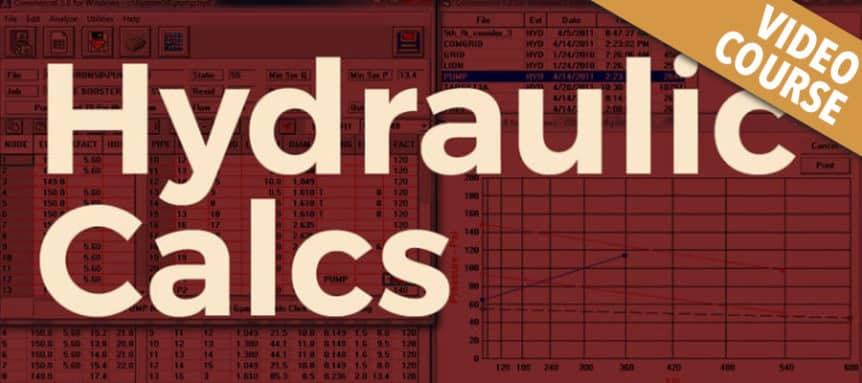# Hydraulic Calcs

Chris

Current Status
Not Enrolled
Price
\$105
Get Started

### Course Description:

This interactive seminar covers the requirements for properly hydraulically calculating a water-based fire protection system in accordance with NFPA-13. The seminar clearly addresses the basic hydraulic formulas utilized, their appropriate application, and various modifications to the calcs required or allowed by the NFPA-13 standards. We will cover and address the various hydraulics tables used in the standard, their specific purposes, and when to use which tables based on system type and application. The attendees will be involved in a significant number of exercises addressing various problems encountered in hydraulic calculation, how to identify when there is a problem with the hydraulics and learn various methods on correcting the identified problems in the calcs. Learning outcomes: At the conclusion of this seminar the participant will be able to:

1. Explain the definitions and equations relative to the hydraulic calculation process.
2. Explain the basis hydraulic principles at play.
3. Explain and perform the basic hydraulic calculation process.
4. Review hydraulic calculations for accuracy and completeness.
5. Understand the basic hydraulic laws of hydrostatics & hydrokinetics.
6. Discuss the various ways flow and pressure act upon the piping and pipe size.
7. Discuss and explain static and residual pressures and the difference between them.
8. Apply the various tables in the standard to the appropriate situation.
9. Explain the effect of elevation pressure to the hydraulic calculations process.
10. Properly calculate velocity pressures created by water moving thru pipes.
11. Understand how the flow of water affects/is affected by the velocity (speed) of the flow.
12. Calculate a small sprinkler branch-line by hand.
13. Understand the primary path of water in a set of hydraulic calculations.
14. Properly review a complete set of hydraulic calculations.

3.0 Hour CE – Technical
Instructor: Ray Vance

### This course is approved for:

• Fire Protection System Contractor I, II
• Fire Protection System Contractor IV
• FL Fire Safety Inspector 1